Question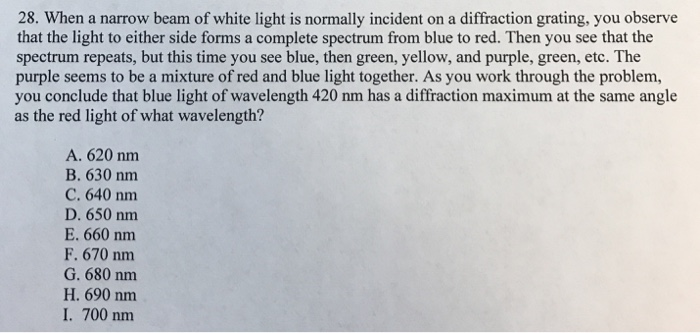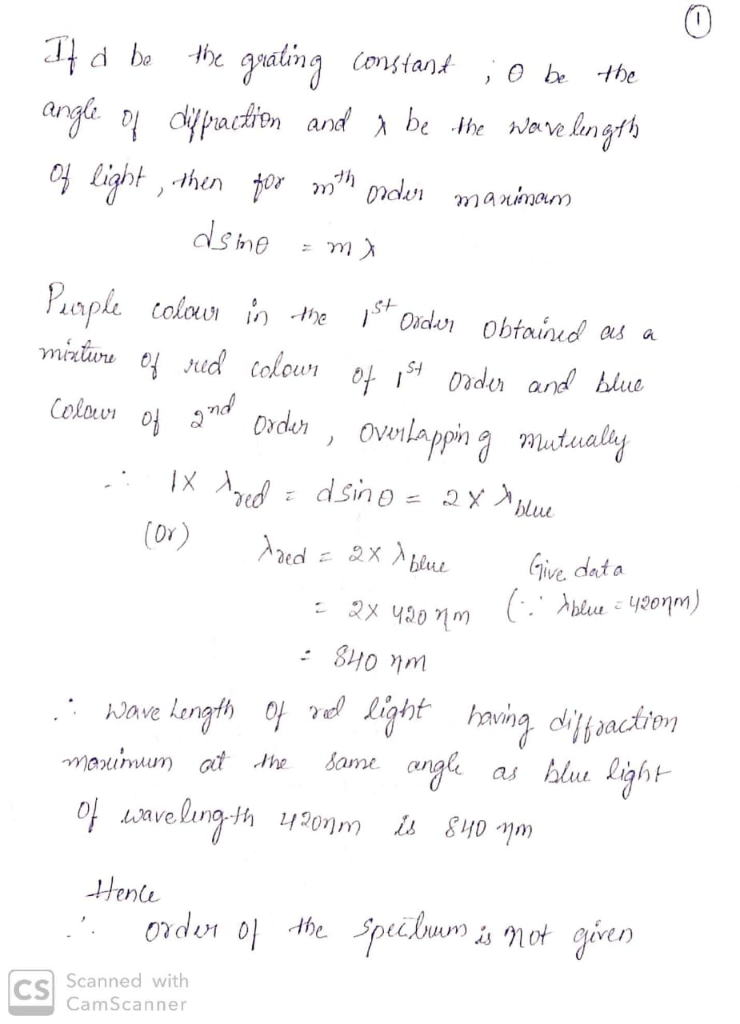#### Earn Coins

Coins can be redeemed for fabulous gifts.

Similar Homework Help Questions
• ### (a) White light is spread out into its spectral components by a diffraction grating. If the...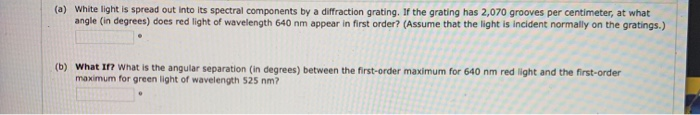(a) White light is spread out into its spectral components by a diffraction grating. If the grating has 2,070 grooves per centimeter, at what angle (in degrees) does red light of wavelength 640 nm appear in first order? (Assume that the light is incident normally on the gratings.) (b) What If? What is the angular separation (in degrees) between the first-order maximum for 640 nm red light and the first-order maximum for green light of wavelength 525 nm? 11. (-/1...

• ### A beam of white light is incident on a diffraction grating which reflects light onto a...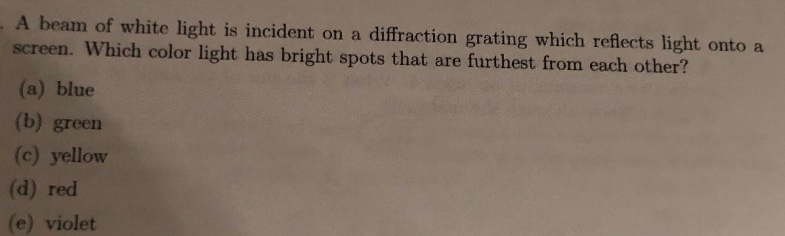A beam of white light is incident on a diffraction grating which reflects light onto a screen. Which color light has bright spots that are furthest from each other? (a) blue (b) green (c) yellow (d) red (e) violet

• ### White light is spread out into its spectral components by a diffraction grating. If the grating...White light is spread out into its spectral components by a diffraction grating. If the grating has 1 970 grooves per centimeter, at what angle does red light of wavelength 640 nm appear in first order? (Assume that the light is incident normally on the gratings.) Mood Holn Bond It Montorit

• ### White light is incident on a diffraction grating with 479 lines/mm. HINT (a) Calculate the angle...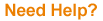White light is incident on a diffraction grating with 479 lines/mm. HINT (a) Calculate the angle θr2 (in degrees) to the second-order maximum for a wavelength of 620 nm. ° (b) Calculate the wavelength in nm of light with a third-order maximum at the same angle θr2. nm

• ### White light is spread out into its spectral components by a diffraction grating

White light is spread out into its spectral components by a diffraction grating. If the grating has 1960 grooves per centimeter, at what angle does red light of wavelength 640 nm appear in first order? (Assume that the light is incident normally on the gratings.)

• ### White light is spread out into its spectral components by a diffraction grating

White light is spread out into its spectral components by a diffraction grating. If the grating has 2000 lines per centimeter, at what angle does red light of wavelength 640 nm appear in first-order spectrum? (Assume that the light is incident normally on the grating.) Unpolarized light passes through two Polaroid sheets. The transmission axis of the analyzer makes an angle of 43.4° with the axis of the polarizer. (a) What fraction of the original unpolarized light is transmitted through the analyzer?...

• ### Intense white light is incident on a diffraction grating that has 460 lines/mm. (a) What is...

Intense white light is incident on a diffraction grating that has 460 lines/mm. (a) What is the highest order in which the complete visible spectrum can be seen with this grating? (Enter 1 for first order, 2 for second order, etc.) (b) What is the angular separation between the violet edge (400 nm) and the red edge (700 nm) of the first order spectrum produced by the grating?

• ### 17. Red light of wavelength 7.00 x 10-7 m, incident normally on a diffraction grating, gave...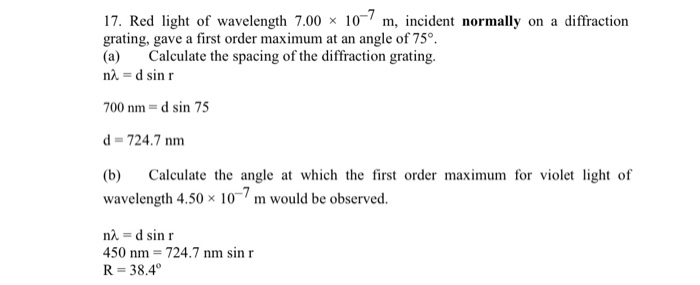17. Red light of wavelength 7.00 x 10-7 m, incident normally on a diffraction grating, gave a first order maximum at an angle of 75°. Calculate the spacing of the diffraction grating. (a) na = d sin r 700 nm = d sin 75 d = 724.7 nm (b) Calculate the angle at which the first order maximum for violet light of wavelength 4.50 x 10-7 m would be observed. na = d sin r 450 nm = 724.7 nm...

• ### Light of wavelength 500 nm is incident normally on a diffraction grating. The third-order maximum of...

Light of wavelength 500 nm is incident normally on a diffraction grating. The third-order maximum of the diffraction pattern is observed at 32.0°. (a) What is the number of rulings per centimeter for the grating?_________grooves/cm (b) Determine the total number of primary maxima that can be observed in this situation.__________

• ### Light of wavelength 500 nm is incident normally on a diffraction grating. The third-order maximum of...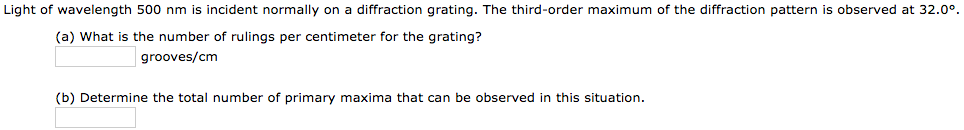Light of wavelength 500 nm is incident normally on a diffraction grating. The third-order maximum of the diffraction pattern is observed at 32.0°. (a) What is the number of rulings per centimeter for the grating? grooves/cm (b) Determine the total number of primary maxima that can be observed in this situation.